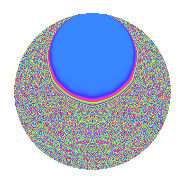# Properties

 Label 3.26.a.aLevel 3 Weight 26 Character orbit 3.a Self dual Yes Analytic conductor 11.880 Analytic rank 1 Dimension 2 CM No Inner twists 1

# Related objects

## Newspace parameters

 Level: $$N$$ = $$3$$ Weight: $$k$$ = $$26$$ Character orbit: $$[\chi]$$ = 3.a (trivial)

## Newform invariants

 Self dual: Yes Analytic conductor: $$11.8799033986$$ Analytic rank: $$1$$ Dimension: $$2$$ Coefficient field: $$\Q(\sqrt{1287001})$$ Coefficient ring: $$\Z[a_1, a_2]$$ Coefficient ring index: $$2^{2}\cdot 3$$ Fricke sign: $$1$$ Sato-Tate group: $\mathrm{SU}(2)$

## $q$-expansion

Coefficients of the $$q$$-expansion are expressed in terms of $$\beta = 6\sqrt{1287001}$$. We also show the integral $$q$$-expansion of the trace form.

 $$f(q)$$ $$=$$ $$q + ( -162 - \beta ) q^{2} -531441 q^{3} + ( 12803848 + 324 \beta ) q^{4} + ( 285430878 + 22912 \beta ) q^{5} + ( 86093442 + 531441 \beta ) q^{6} + ( -14843692864 + 5152896 \beta ) q^{7} + ( -11649985056 + 20698096 \beta ) q^{8} + 282429536481 q^{9} +O(q^{10})$$ $$q +(-162 - \beta) q^{2} -531441 q^{3} +(12803848 + 324 \beta) q^{4} +(285430878 + 22912 \beta) q^{5} +(86093442 + 531441 \beta) q^{6} +(-14843692864 + 5152896 \beta) q^{7} +(-11649985056 + 20698096 \beta) q^{8} +282429536481 q^{9} +(-1107799411068 - 289142622 \beta) q^{10} +(-9093892809276 - 983057152 \beta) q^{11} +(-6804489784968 - 172186884 \beta) q^{12} +(-52571818339618 + 8456327424 \beta) q^{13} +(-236339484732288 + 14008923712 \beta) q^{14} +(-151689671235198 - 12176376192 \beta) q^{15} +(-1386723478478720 - 2574742464 \beta) q^{16} +(-228493682174718 - 310973856000 \beta) q^{17} +(-45753584909922 - 282429536481 \beta) q^{18} +(6948719247673532 + 1780077627648 \beta) q^{19} +(3998558889680112 + 385841369848 \beta) q^{20} +(7888546979337024 - 2738460203136 \beta) q^{21} +(47020249991624184 + 9253148067900 \beta) q^{22} +(69671149450176888 - 20198378016512 \beta) q^{23} +(6191279708145696 - 10999816836336 \beta) q^{24} +(-192229984003543457 + 13079584553472 \beta) q^{25} +(-383282232065537148 + 51201893296930 \beta) q^{26} -150094635296999121 q^{27} +(-112703278385033728 + 61167540655872 \beta) q^{28} +(-1251016745130354906 - 126217146058624 \beta) q^{29} +(588730026817388988 + 153662244178302 \beta) q^{30} +(537736051246227656 - 1052676722058624 \beta) q^{31} +(734850895408897536 + 692627731996416 \beta) q^{32} +(4832867488454446716 + 522436875916032 \beta) q^{33} +(14445067867763120316 + 278871446846718 \beta) q^{34} +(1233257975178122880 + 1130696938622720 \beta) q^{35} +(3616184855813178888 + 91507169819844 \beta) q^{36} +(-985735474619156314 + 295504419926016 \beta) q^{37} +(-83600313245104843512 - 7237091823352508 \beta) q^{38} +(27938819710224929538 - 4494039102537984 \beta) q^{39} +(18646997230106224704 + 5640951256605216 \beta) q^{40} +(-$$$$20\!\cdots\!34$$$$+ 5221410776755456 \beta) q^{41} +($$$$12\!\cdots\!08$$$$- 7444916426428992 \beta) q^{42} +(19465197949129913540 + 45176076103631616 \beta) q^{43} +(-$$$$13\!\cdots\!76$$$$- 15533335619726320 \beta) q^{44} +(80614110570904860318 + 6471025539852672 \beta) q^{45} +($$$$92\!\cdots\!76$$$$- 66399012211501944 \beta) q^{46} +(-$$$$36\!\cdots\!20$$$$- 9934991015089408 \beta) q^{47} +($$$$73\!\cdots\!20$$$$+ 1368323709810624 \beta) q^{48} +($$$$10\!\cdots\!65$$$$- 152976011168268288 \beta) q^{49} +(-$$$$57\!\cdots\!58$$$$+ 190111091305880993 \beta) q^{50} +($$$$12\!\cdots\!38$$$$+ 165264257006496000 \beta) q^{51} +(-$$$$54\!\cdots\!28$$$$+ 91240261833091320 \beta) q^{52} +(-$$$$42\!\cdots\!82$$$$+ 15631770768985728 \beta) q^{53} +(24315330918113857602 + 150094635296999121 \beta) q^{54} +(-$$$$36\!\cdots\!92$$$$- 488954138065671168 \beta) q^{55} +($$$$51\!\cdots\!60$$$$- 367267341288709120 \beta) q^{56} +(-$$$$36\!\cdots\!12$$$$- 946006234514880768 \beta) q^{57} +($$$$60\!\cdots\!36$$$$+ 1271463922791851994 \beta) q^{58} +($$$$42\!\cdots\!48$$$$- 397484438615856128 \beta) q^{59} +(-$$$$21\!\cdots\!92$$$$- 205051923433390968 \beta) q^{60} +(-$$$$12\!\cdots\!30$$$$+ 2464520538925757952 \beta) q^{61} +($$$$48\!\cdots\!92$$$$- 367202422272730568 \beta) q^{62} +(-$$$$41\!\cdots\!84$$$$+ 1455330028814798976 \beta) q^{63} +($$$$14\!\cdots\!32$$$$- 760662567066516480 \beta) q^{64} +(-$$$$60\!\cdots\!36$$$$+ 1209171459490470656 \beta) q^{65} +(-$$$$24\!\cdots\!44$$$$- 4917502262352843900 \beta) q^{66} +(-$$$$67\!\cdots\!28$$$$- 7885549958339957760 \beta) q^{67} +(-$$$$75\!\cdots\!64$$$$- 4055693937222496632 \beta) q^{68} +(-$$$$37\!\cdots\!08$$$$+ 10734246211473153792 \beta) q^{69} +(-$$$$52\!\cdots\!80$$$$- 1416430879235003520 \beta) q^{70} +($$$$60\!\cdots\!92$$$$+ 18643323271852842240 \beta) q^{71} +(-$$$$32\!\cdots\!36$$$$+ 5845753659319240176 \beta) q^{72} +($$$$42\!\cdots\!38$$$$- 13385360579839137792 \beta) q^{73} +(-$$$$13\!\cdots\!08$$$$+ 937863758591141722 \beta) q^{74} +($$$$10\!\cdots\!37$$$$- 6951027494681713152 \beta) q^{75} +($$$$11\!\cdots\!08$$$$+ 25043228408851813872 \beta) q^{76} +(-$$$$99\!\cdots\!48$$$$- 32267685449300499968 \beta) q^{77} +($$$$20\!\cdots\!68$$$$- 27210785375613776130 \beta) q^{78} +($$$$63\!\cdots\!40$$$$- 27385251957433054080 \beta) q^{79} +(-$$$$39\!\cdots\!08$$$$- 32507519341027836032 \beta) q^{80} +$$$$79\!\cdots\!61$$$$q^{81} +(-$$$$20\!\cdots\!08$$$$+$$$$20\!\cdots\!62$$$$\beta) q^{82} +(-$$$$43\!\cdots\!56$$$$+ 42027911871325874432 \beta) q^{83} +($$$$59\!\cdots\!48$$$$- 32506938973697271552 \beta) q^{84} +(-$$$$39\!\cdots\!04$$$$- 93996787999112706816 \beta) q^{85} +(-$$$$20\!\cdots\!56$$$$- 26783722277918235332 \beta) q^{86} +($$$$66\!\cdots\!46$$$$+ 67076966318541197184 \beta) q^{87} +(-$$$$83\!\cdots\!56$$$$-$$$$17\!\cdots\!84$$$$\beta) q^{88} +($$$$10\!\cdots\!22$$$$+$$$$13\!\cdots\!68$$$$\beta) q^{89} +(-$$$$31\!\cdots\!08$$$$- 81662416708360993182 \beta) q^{90} +($$$$27\!\cdots\!96$$$$-$$$$39\!\cdots\!64$$$$\beta) q^{91} +($$$$58\!\cdots\!56$$$$-$$$$23\!\cdots\!64$$$$\beta) q^{92} +(-$$$$28\!\cdots\!96$$$$+$$$$55\!\cdots\!84$$$$\beta) q^{93} +($$$$52\!\cdots\!28$$$$+$$$$37\!\cdots\!16$$$$\beta) q^{94} +($$$$38\!\cdots\!32$$$$+$$$$66\!\cdots\!28$$$$\beta) q^{95} +(-$$$$39\!\cdots\!76$$$$-$$$$36\!\cdots\!56$$$$\beta) q^{96} +(-$$$$27\!\cdots\!58$$$$- 71593944273965145600 \beta) q^{97} +($$$$70\!\cdots\!38$$$$- 84708326791237796409 \beta) q^{98} +(-$$$$25\!\cdots\!56$$$$-$$$$27\!\cdots\!12$$$$\beta) q^{99} +O(q^{100})$$ $$\operatorname{Tr}(f)(q)$$ $$=$$ $$2q - 324q^{2} - 1062882q^{3} + 25607696q^{4} + 570861756q^{5} + 172186884q^{6} - 29687385728q^{7} - 23299970112q^{8} + 564859072962q^{9} + O(q^{10})$$ $$2q - 324q^{2} - 1062882q^{3} + 25607696q^{4} + 570861756q^{5} + 172186884q^{6} - 29687385728q^{7} - 23299970112q^{8} + 564859072962q^{9} - 2215598822136q^{10} - 18187785618552q^{11} - 13608979569936q^{12} - 105143636679236q^{13} - 472678969464576q^{14} - 303379342470396q^{15} - 2773446956957440q^{16} - 456987364349436q^{17} - 91507169819844q^{18} + 13897438495347064q^{19} + 7997117779360224q^{20} + 15777093958674048q^{21} + 94040499983248368q^{22} + 139342298900353776q^{23} + 12382559416291392q^{24} - 384459968007086914q^{25} - 766564464131074296q^{26} - 300189270593998242q^{27} - 225406556770067456q^{28} - 2502033490260709812q^{29} + 1177460053634777976q^{30} + 1075472102492455312q^{31} + 1469701790817795072q^{32} + 9665734976908893432q^{33} + 28890135735526240632q^{34} + 2466515950356245760q^{35} + 7232369711626357776q^{36} - 1971470949238312628q^{37} -$$$$16\!\cdots\!24$$$$q^{38} + 55877639420449859076q^{39} + 37293994460212449408q^{40} -$$$$41\!\cdots\!68$$$$q^{41} +$$$$25\!\cdots\!16$$$$q^{42} + 38930395898259827080q^{43} -$$$$26\!\cdots\!52$$$$q^{44} +$$$$16\!\cdots\!36$$$$q^{45} +$$$$18\!\cdots\!52$$$$q^{46} -$$$$73\!\cdots\!40$$$$q^{47} +$$$$14\!\cdots\!40$$$$q^{48} +$$$$21\!\cdots\!30$$$$q^{49} -$$$$11\!\cdots\!16$$$$q^{50} +$$$$24\!\cdots\!76$$$$q^{51} -$$$$10\!\cdots\!56$$$$q^{52} -$$$$84\!\cdots\!64$$$$q^{53} + 48630661836227715204q^{54} -$$$$72\!\cdots\!84$$$$q^{55} +$$$$10\!\cdots\!20$$$$q^{56} -$$$$73\!\cdots\!24$$$$q^{57} +$$$$12\!\cdots\!72$$$$q^{58} +$$$$84\!\cdots\!96$$$$q^{59} -$$$$42\!\cdots\!84$$$$q^{60} -$$$$24\!\cdots\!60$$$$q^{61} +$$$$97\!\cdots\!84$$$$q^{62} -$$$$83\!\cdots\!68$$$$q^{63} +$$$$28\!\cdots\!64$$$$q^{64} -$$$$12\!\cdots\!72$$$$q^{65} -$$$$49\!\cdots\!88$$$$q^{66} -$$$$13\!\cdots\!56$$$$q^{67} -$$$$15\!\cdots\!28$$$$q^{68} -$$$$74\!\cdots\!16$$$$q^{69} -$$$$10\!\cdots\!60$$$$q^{70} +$$$$12\!\cdots\!84$$$$q^{71} -$$$$65\!\cdots\!72$$$$q^{72} +$$$$84\!\cdots\!76$$$$q^{73} -$$$$27\!\cdots\!16$$$$q^{74} +$$$$20\!\cdots\!74$$$$q^{75} +$$$$23\!\cdots\!16$$$$q^{76} -$$$$19\!\cdots\!96$$$$q^{77} +$$$$40\!\cdots\!36$$$$q^{78} +$$$$12\!\cdots\!80$$$$q^{79} -$$$$79\!\cdots\!16$$$$q^{80} +$$$$15\!\cdots\!22$$$$q^{81} -$$$$41\!\cdots\!16$$$$q^{82} -$$$$87\!\cdots\!12$$$$q^{83} +$$$$11\!\cdots\!96$$$$q^{84} -$$$$79\!\cdots\!08$$$$q^{85} -$$$$41\!\cdots\!12$$$$q^{86} +$$$$13\!\cdots\!92$$$$q^{87} -$$$$16\!\cdots\!12$$$$q^{88} +$$$$21\!\cdots\!44$$$$q^{89} -$$$$62\!\cdots\!16$$$$q^{90} +$$$$55\!\cdots\!92$$$$q^{91} +$$$$11\!\cdots\!12$$$$q^{92} -$$$$57\!\cdots\!92$$$$q^{93} +$$$$10\!\cdots\!56$$$$q^{94} +$$$$77\!\cdots\!64$$$$q^{95} -$$$$78\!\cdots\!52$$$$q^{96} -$$$$55\!\cdots\!16$$$$q^{97} +$$$$14\!\cdots\!76$$$$q^{98} -$$$$51\!\cdots\!12$$$$q^{99} + O(q^{100})$$

## Embeddings

For each embedding $$\iota_m$$ of the coefficient field, the values $$\iota_m(a_n)$$ are shown below.

For more information on an embedded modular form you can click on its label.

Label $$\iota_m(\nu)$$ $$a_{2}$$ $$a_{3}$$ $$a_{4}$$ $$a_{5}$$ $$a_{6}$$ $$a_{7}$$ $$a_{8}$$ $$a_{9}$$ $$a_{10}$$
1.1
 567.730 −566.730
−6968.76 −531441. 1.50092e7 4.41387e8 3.70349e9 2.02309e10 1.29237e11 2.82430e11 −3.07592e12
1.2 6644.76 −531441. 1.05985e7 1.29474e8 −3.53130e9 −4.99182e10 −1.52537e11 2.82430e11 8.60326e11
 $$n$$: e.g. 2-40 or 990-1000 Significant digits: Format: Complex embeddings Normalized embeddings Satake parameters Satake angles

## Inner twists

This newform does not admit any (nontrivial) inner twists.

## Atkin-Lehner signs

$$p$$ Sign
$$3$$ $$1$$

## Hecke kernels

This newform can be constructed as the kernel of the linear operator $$T_{2}^{2} + 324 T_{2} - 46305792$$ acting on $$S_{26}^{\mathrm{new}}(\Gamma_0(3))$$.